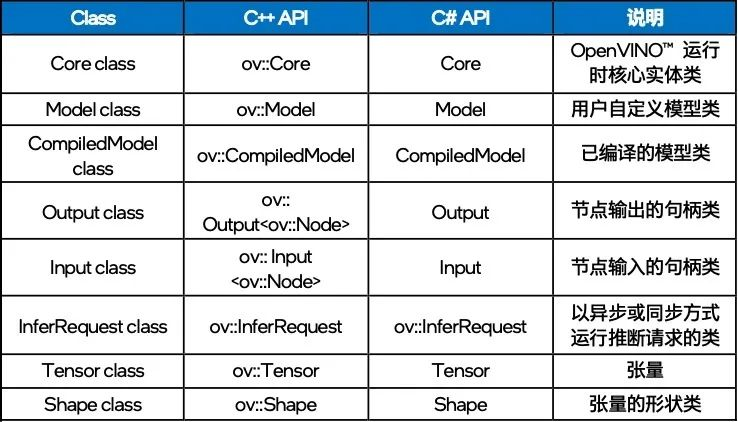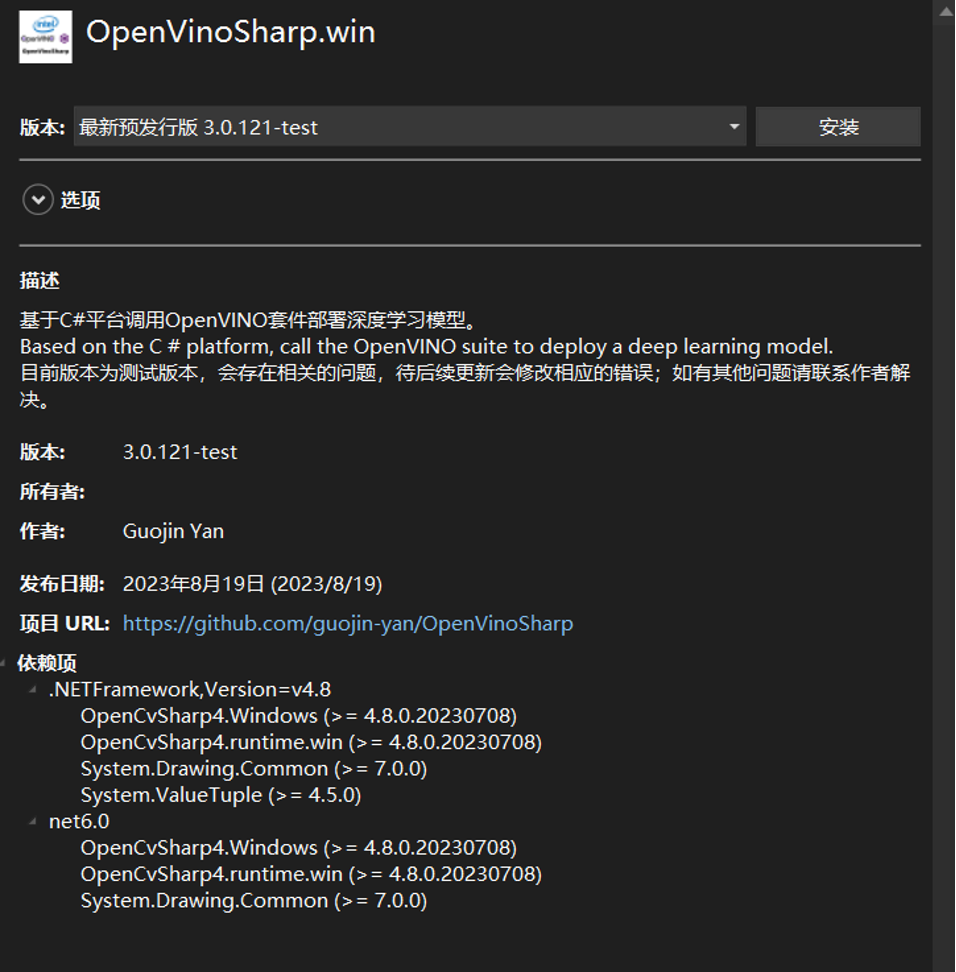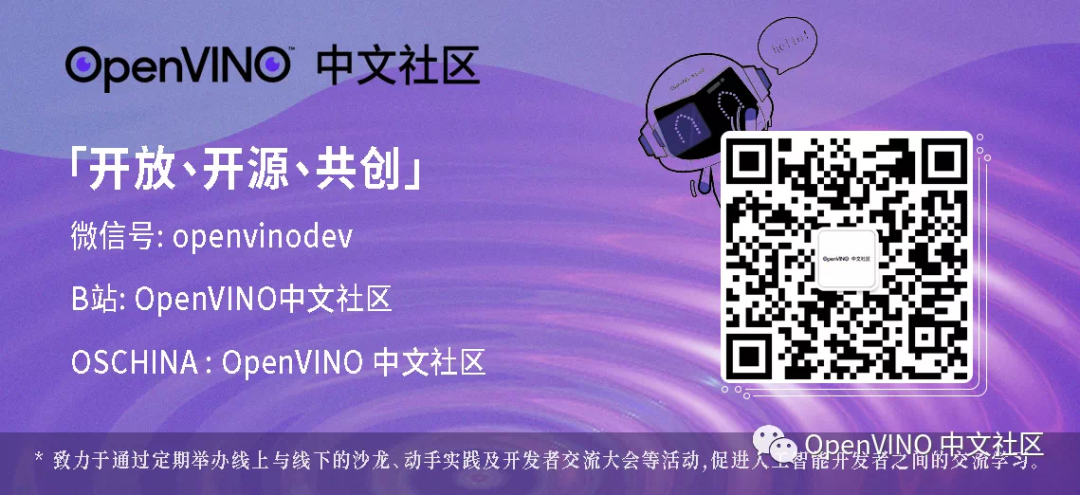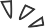# 开发者实战 | OpenVINOSharp 常用 API 详解与演示

09/05 20:00OpenVINO™ 工具套件可以加快深度学习视觉应用开发速度，帮助用户在从边缘到云的各种英特尔平台上，更加方便快捷的将 AI 模型部署到生产系统中。

C# 是由 C 和 C++ 衍生出来的一种安全的、稳定的、简单的、优雅的面向对象编程语言，它综合了 VB 简单的可视化操作和 C++ 的高运行效率，成为支持成为.NET 开发的首选语言。作为人工智能开发人员，如果你希望在 C# 端使用 OpenVINO™ ，OpenVINOSharp 将是你的首选，并且制作了 NuGet 程序包，实现在 C# 端了一站式安装与使用 OpenVINO™

OpenVINOSharp GitHub: https://github.com/guojin-yan/OpenVinoSharp

OpenVINOSharp 在制作时参考了 OpenVINO™ C++ API，因此对于之前使用过 OpenVINO™ 的人十分友好。下面表格向我们展示了 C# 与 C++ API 的对应关系1.1

OpenVINOSharp 支持 NuGet 程序包安装方式，这与在 C++ 中安装过程相比，较为简单，并且程序包中包含了 OpenVINO™ 2023.0 发行版本，可以通过 NuGet 安装后直接使用。dotnet add package OpenVinoSharp.win

1.2

OpenVINOSharp 程序集全部在命名空间 OpenVinoSharp 下，因此若要使用 OpenVINOSharp ，需要先引入命名空间：

using OpenVinoSharp

1.3

Core 类代表一个 OpenVINO™ 运行时核心实体，后续的读取模型、加载模型等方法都需要通过 Core 类进行创建，在封装 C# API 时，为了与 C++ API 对应，也对 Core 类进行了封装，并封装了与 C++ API 中对应的方法。

Core core = new Core()

ov::Core core;

1.4

1.4.1   加载模型

OpenVINO™ 2022.1版本更新之后，加载，下面是所使用的 API 方法：

Model model = core.read_model(model_path);

std::shared_ptrmodel = core.read_model(model_path);

1.4.1   获取模型信息

 API 作用 Model.get_friendly_name() 获取模型的friendly name。 Model.input() 获取模型的输入层，并返回 Input 对象。 Model.output() 获取模型的输出层，并返回 Output 对象。

Input/Output 主要是封装了模型网络层，可以通过下面 API 实现获取模型的详细信息：

 API 作用 Output.get_any_name() 获取模型网络层的名字。 Output.get_element_type() 获取模型网络层的数据类型，并返回 OvType 对象，OvType 主要封装了网络的基本数据类型。 Output.get_shape() 获取模型网络层的形状，并返回 Shape 对象，Shape 封装了网络层的形状数组。

string model_name = model.get_friendly_name();Input input = model.input();Output output = model.output();

Console.WriteLine("Model name: {0}", model_name);Console.WriteLine("/------- [In] -------/");Console.WriteLine("Input name: {0}", input.get_any_name());Console.WriteLine("Input type: {0}", input.get_element_type().to_string());Console.WriteLine("Input shape: {0}", input.get_shape().to_string());Console.WriteLine("/------- [Out] -------/");Console.WriteLine("Output name: {0}", output.get_any_name());Console.WriteLine("Output type: {0}", output.get_element_type().to_string());Console.WriteLine("Output shape: {0}", output.get_shape().to_string());

Model name: torch_jit/------- [In] -------/Input name: dataInput type: floatInput shape: [1,3,224,224]/------- [Out] -------/Output name: probOutput type: floatOutput shape: [1,1000]

std::cout << "Model name: " << model->get_friendly_name() << std::endl;ov::Output<ov::Node> input = model->input();std::cout << "/------- [In] -------/" << std::endl;std::cout << "Input name: " << input.get_any_name() << std::endl;std::cout << "Input type: " << input.get_element_type().c_type_string() << std::endl;std::cout << "Input shape: " << input.get_shape().to_string() << std::endl;ov::Output<ov::Node> output = model->output();std::cout << "/------- [Out] -------/" << std::endl;std::cout << "Output name: " << output.get_any_name() << std::endl;std::cout << "Output type: " << output.get_element_type().c_type_string() << std::endl;std::cout << "Output shape: " << output.get_shape().to_string() << std::endl;

1.5

 API 作用 Core.compile_model() 将模型编译为可以在目标设备上执行的 compile_model 对象。 CompiledModel.create_infer_request() 创建用于推断已编译模型的推断请求对象，创建的请求已经分配了输入和输出张量。

CompiledModel compiled_model = core.compile_model(model, "AUTO");InferRequest infer_request = compiled_model.create_infer_request();

CompiledModel compiled_model = core.compile_model(model, "AUTO");InferRequest infer_request = compiled_model.create_infer_request();

1.6

1.6.1   张量的获取与设置

 API 作用 InferRequest.set_tensor() 设置要推断的输入/输出张量。 InferRequest.set_input_tensor() 设置要推断的输入张量。 InferRequest.set_output_tensor() 设置要推断的输出张量 InferRequest.get_tensor() 获取用于推理的输入/输出张量。 InferRequest.get_input_tensor() 获取用于推理的输入张量。 InferRequest.get_output_tensor() 设置要推理的输出张量

1.6.2   张量的信息获取与设置

 API 作用 Tensor.set_shape () 给张量设置一个新的形状。 Tensor.get_shape() 获取张量的形状。 Tensor.get_element_type() 获取张量的数据类型。 Tensor.get_size() 获取张量的数据长度。 Tensor.get_byte_size() 获取张量的字节大小。 Tensor.data() 获取张量的内存地址。 Tensor.set_data() 将指定类型的数据加载到张量内存下。 Tensor.get_data() 从张量中读取指定类型的数据。

1.7

1.7.1   获取输入张量

Tensor input_tensor = infer_request.get_input_tensor();Console.WriteLine("/------- [Input tensor] -------/");Console.WriteLine("Input tensor type: {0}", input_tensor.get_element_type().to_string());Console.WriteLine("Input tensor shape: {0}", input_tensor.get_shape().to_string());Console.WriteLine("Input tensor size: {0}", input_tensor.get_size());

/------- [Input tensor] -------/Input tensor type: f32Input tensor shape: Shape : {1, 3, 224, 224}Input tensor size: 150528

ov::Tensor input_tensor = infer_request.get_input_tensor();std::cout << "/------- [Input tensor] -------/" << std::endl;std::cout << "Input tensor type: " << input_tensor.get_element_type().c_type_string() << std::endl;std::cout << "Input tensor shape: " << input_tensor.get_shape().to_string() << std::endl;std::cout << "Input tensor size: " << input_tensor.get_size() << std::endl;

1.7.2   添加推理数据

Mat input_mat = new Mat();Shape input_shape = input_tensor.get_shape();long channels = input_shape;long height = input_shape;long width = input_shape;float[] input_data = new float[channels * height * width];Marshal.Copy(input_mat.Ptr(0), input_data, 0, input_data.Length);input_tensor.set_data(input_data);

cv::Mat input_mat；float* input_data = input_tensor.data<float>();ov::Shape input_shape = input_tensor.get_shape();size_t channels = input_shape;size_t height = input_shape;size_t width = input_shape;for (size_t c = 0; c < channels; ++c) {    for (size_t h = 0; h < height; ++h) {        for (size_t w = 0; w < width; ++w) {            input_data[c * height * width + h * width + w] = input_mat.at<cv::Vec<float, 3>>(h, w)[c];        }    }}

1.8

 API 作用 InferRequest.infer() 在同步模式下推断指定的输入。

infer_request.infer();

C++ 中的代码与 C++ 中一致。

1.9

Tensor output_tensor = infer_request.get_output_tensor();Console.WriteLine("/------- [Output tensor] -------/");Console.WriteLine("Output tensor type: {0}", output_tensor.get_element_type().to_string());Console.WriteLine("Output tensor shape: {0}", output_tensor.get_shape().to_string());Console.WriteLine("Output tensor size: {0}", output_tensor.get_size());

/------- [Output tensor] -------/Output tensor type: f32Output tensor shape: Shape : {1, 1000}Output tensor size: 1000

float[] result = output_tensor.get_data<float>(1000);

const float* output_data = output_tensor.data<const float>();float result;for (int i = 0; i < 1000; ++i) {result[i] = *output_data;output_data++;}

1.10

output_tensor.dispose();input_shape.dispose();infer_request.dispose();compiled_model.dispose();input.dispose();output.dispose();model.dispose();core.dispose();

1.11

Yolov8 分类模型示例

using OpenCvSharp;using OpenCvSharp.Dnn;using OpenVinoSharp;using System.Data;using System.Runtime.InteropServices;namespace test_openvinosharp_api{    internal class Program    {        static void Main(string[] args)        {            string model_path = "E:\\GitSpace\\OpenVinoSharp\\model\\yolov8\\yolov8s-cls.xml";            Core core = new Core(); // 初始化推理核心            Model model = core.read_model(model_path); // 读取本地模型            CompiledModel compiled_model = core.compile_model(model, "AUTO"); // 便哟模型到指定设备            // 获取模型的输入输出信息            Console.WriteLine("Model name: {0}", model.get_friendly_name());            Input input = compiled_model.input();            Console.WriteLine("/------- [In] -------/");            Console.WriteLine("Input name: {0}", input.get_any_name());            Console.WriteLine("Input type: {0}", input.get_element_type().to_string());            Console.WriteLine("Input shape: {0}", input.get_shape().to_string());            Output output = compiled_model.output();            Console.WriteLine("/------- [Out] -------/");            Console.WriteLine("Output name: {0}", output.get_any_name());            Console.WriteLine("Output type: {0}", output.get_element_type().to_string());            Console.WriteLine("Output shape: {0}", output.get_shape().to_string());            // 创建推理请求            InferRequest infer_request = compiled_model.create_infer_request();            // 获取输入张量            Tensor input_tensor = infer_request.get_input_tensor();            Console.WriteLine("/------- [Input tensor] -------/");            Console.WriteLine("Input tensor type: {0}", input_tensor.get_element_type().to_string());            Console.WriteLine("Input tensor shape: {0}", input_tensor.get_shape().to_string());            Console.WriteLine("Input tensor size: {0}", input_tensor.get_size());            // 读取并处理输入数据            Mat image = Cv2.ImRead(@"E:\GitSpace\OpenVinoSharp\dataset\image\demo_7.jpg");            Mat input_mat = new Mat();            input_mat = CvDnn.BlobFromImage(image, 1.0 / 255.0, new Size(224, 224), 0, true, false);            // 加载推理数据            Shape input_shape = input_tensor.get_shape();            long channels = input_shape;            long height = input_shape;            long width = input_shape;            float[] input_data = new float[channels * height * width];            Marshal.Copy(input_mat.Ptr(0), input_data, 0, input_data.Length);            input_tensor.set_data(input_data);            // 模型推理            infer_request.infer();             // 获取输出张量            Tensor output_tensor = infer_request.get_output_tensor();            Console.WriteLine("/------- [Output tensor] -------/");            Console.WriteLine("Output tensor type: {0}", output_tensor.get_element_type().to_string());            Console.WriteLine("Output tensor shape: {0}", output_tensor.get_shape().to_string());            Console.WriteLine("Output tensor size: {0}", output_tensor.get_size());            // 获取输出数据            float[] result = output_tensor.get_data<float>(1000);            List<float[]> new_list = new List<float[]> { };            for (int i = 0; i < result.Length; i++)            {                new_list.Add(new float[] { (float)result[i], i });            }            new_list.Sort((a, b) => b.CompareTo(a));            KeyValuePair<int, float>[] cls = new KeyValuePair<int, float>;            for (int i = 0; i < 10; ++i)            {                cls[i] = new KeyValuePair<int, float>((int)new_list[i], new_list[i]);            }            Console.WriteLine("\n Classification Top 10 result : \n");            Console.WriteLine("classid probability");            Console.WriteLine("------- -----------");            for (int i = 0; i < 10; ++i)            {                Console.WriteLine("{0}     {1}", cls[i].Key.ToString("0"), cls[i].Value.ToString("0.000000"));            }            // 销毁非托管内存            output_tensor.dispose();            input_shape.dispose();            infer_request.dispose();            compiled_model.dispose();            input.dispose();            output.dispose();            model.dispose();            core.dispose();        }    }}

1.12

--END--



你也许想了解(点击蓝字查看)⬇️

➡️

【硬核集锦】2023英特尔学术大会限定放送

➡️ 基于 ChatGLM2 和 OpenVINO™ 打造中文聊天助手

➡️ 基于 Llama2 和 OpenVINO™ 打造聊天机器人

➡️ OpenVINO™ DevCon 2023重磅回归！英特尔以创新产品激发开发者无限潜能

➡️ 5周年更新 | OpenVINO™  2023.0，让AI部署和加速更容易

➡️ OpenVINO™5周年重头戏！2023.0版本持续升级AI部署和加速性能

➡️ OpenVINO™2023.0实战 | 在 LabVIEW 中部署 YOLOv8 目标检测模型

➡️ 开发者实战系列资源包来啦！

➡️

以AI作画，祝她节日快乐；简单三步，OpenVINO™ 助你轻松体验AIGC

➡️

还不知道如何用OpenVINO™作画？点击了解教程。

➡️

➡️

使用OpenVINO 在“端—边—云”快速实现高性能人工智能推理

➡️

图片提取文字很神奇？试试三步实现OCR！

➡️

【Notebook系列第六期】基于Pytorch预训练模型，实现语义分割任务

➡️

使用OpenVINO™ 预处理API进一步提升YOLOv5推理性能





扫描下方二维码立即体验
OpenVINO™ 工具套件 2023.00 评论
0 收藏
0